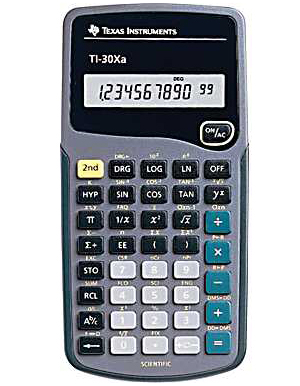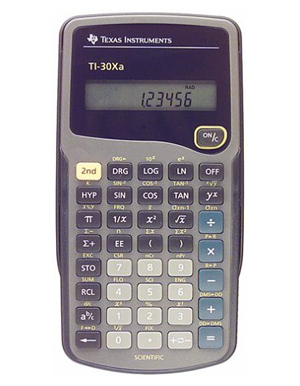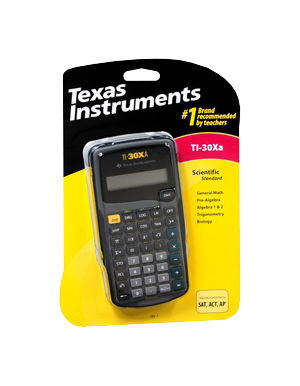Calculator BrandsTI 30XA Scientific Calculator

Product Features
• Adds, subtracts, multiplies, and divides fractions entered in traditional numerator/denominator format
• Performs trigonometric functions, logarithms, roots, powers, reciprocals, and factorials
• Polar/rectangular conversions
• 1-variable statistics include results for mean and standard deviation
• Battery powered; slide case included

Product Description

Texas Instruments scientific calculator with 10-digit display performs basic scientific and trigonometric functions. Adds, subtracts, multiplies and divides fractions entered in traditional numerator/denominator format. Automatic power down. Other features include: one-variable statistics; trig functions in degrees, radians, grads and hyperbolics; common and natural algorithms; universal powers and roots, reciprocals and factorials; polar and rectangular conversions. Also includes protective case, guidebook and 2 A76 button cell batteries. No. TI-30XA. Imported.

Price : \$19.99LINKSDISCLAIMERCONTACT US